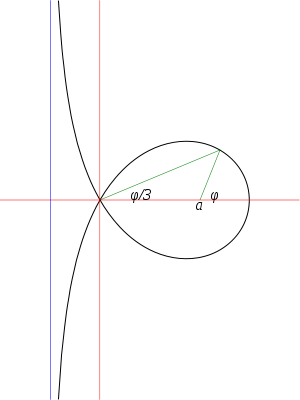# Trisectrix of MaclaurinThe Trisectrix of Maclaurin showing the angle trisection property

In geometry, the trisectrix of Maclaurin is a cubic plane curve notable for its trisectrix property, meaning it can be used to trisect an angle. It can be defined as locus of the point of intersection of two lines, each rotating at a uniform rate about separate points, so that the ratio of the rates of rotation is 1:3 and the lines initially coincide with the line between the two points. A generalization of this construction is called a sectrix of Maclaurin. The curve is named after Colin Maclaurin who investigated the curve in 1742.

## Equations

Let two lines rotate about the pointsandso that when the line rotating abouthas anglewith the x axis, the rotating abouthas angle. Letbe the point of intersection, then the angle formed by the lines atis. By the law of sines,so the equation in polar coordinates is (up to translation and rotation).

The curve is therefore a member of the Conchoid of de Sluze family.

In Cartesian coordinates the equation of this curve is.

If the origin is moved to (a, 0) then a derivation similar to that given above shows that the equation of the curve in polar coordinates becomesmaking it an example of an epispiral.

## The trisection property

Given an angle, draw a ray fromwhose angle with the-axis is. Draw a ray from the origin to the point where the first ray intersects the curve. Then, by the construction of the curve, the angle between the second ray and the-axis is## Notable points and features

The curve has an x-intercept atand a double point at the origin. The vertical lineis an asymptote. The curve intersects the line x = a, or the point corresponding to the trisection of a right angle, at. As a nodal cubic, it is of genus zero.

## Relationship to other curves

The trisectrix of Maclaurin can be defined from conic sections in three ways. Specifically:.and the linerelative to the origin..